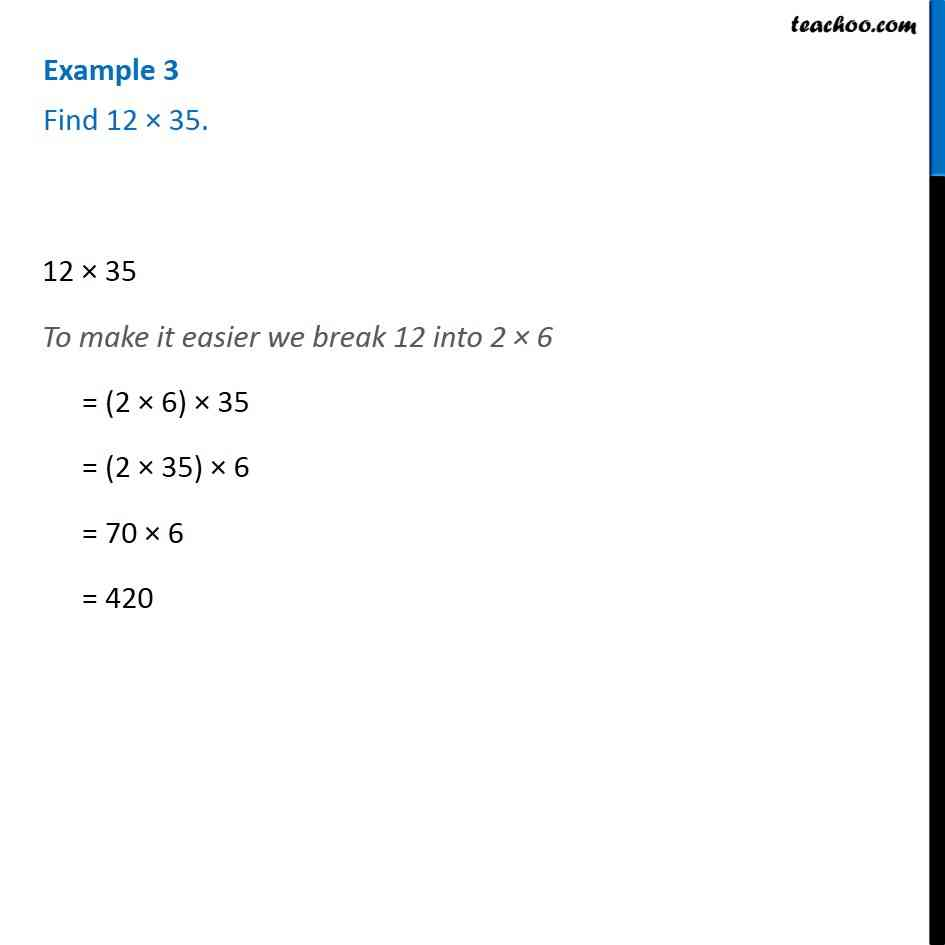1. Chapter 2 Class 6 Whole Numbers
2. Concept wise
3. Multiplication and Addition of Whole numbers

Transcript

Example 3 - Chapter 2 Class 6 Whole Numbers - NCERT Book Find 12 × 35. 12 × 35 To make it easier we break 12 into 2 6 = (2 × 6) 35 = (2 × 35) 6 = 70 × 6 = 420

Multiplication and Addition of Whole numbers